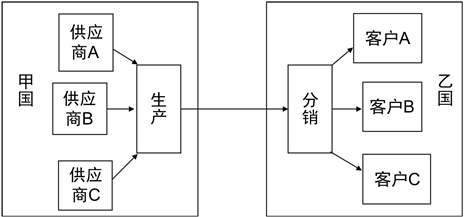# 贸易品的家乡偏好之谜综述Review of the Puzzle of Home Bias in Trade

DOI: 10.12677/ASS.2021.107261, PDF, HTML, XML, 下载: 21  浏览: 46

Abstract: The Puzzle of Home Bias in Trade is among the famous “6 Puzzles” in International Macroeconomy. From the 1990s, the study of this puzzle continues. Many scholars had given their own explanations of this puzzle. This article is a literature review of The Puzzle of Home Bias in the past 30 years. And it discussed the reason, the economic models and possible explanations of the puzzle. The conclusion is that home bias does exist and relates to the trade costs, multi-stage production supply chain and consumers preference bias. There are differences between various trade goods. In the end, this article emphasizes the test of some typical views empirically, summarizes the researches, and comes up with some policy enlightens.

1. 谜题的起源

1994年NAFTA生效后，John Mccallum (1995，以下简称J.M.)在研究美国和加拿大区域贸易时发现了两个反常的现象：1) 美国和加拿大的国内贸易额远大于美加间贸易额。2) 加拿大省份间贸易额是加拿大同美国各州间贸易额的约20倍 。考虑到1年以内，NAFTA的作用可能尚未完全起效，Helliwell (1996, 1998)在后续研究加拿大和美国的边界效应时，发现加拿大省份之间贸易额同加拿大美国间的贸易额之比虽然从20减少到了12，但是依然很明显 。Shang-jin Wei (1996)在研究OECD内贸的时候也发现了上述现象 ；Nitsch (2000)在研究欧盟内贸的时候也发现了类似的现象 。众多现象表明：贸易的确存在家乡偏好：本国的贸易量的确是多于对外贸易的贸易量。

2. 建立模型

2.1. CES模型的构建和比较静态分析

Maurice Obstfeld和Kenneth Rogoff (2001)通过构建CES函数并引入价格约束机制的方式来建立一般均衡模型，并使用比较静态分析的方法得到关于偏好影响机制的均衡解，并最后分析出影响贸易本地偏好的影响因素 。

$C={\left({C}_{H}^{\frac{\theta -1}{\theta }}+{C}_{F}^{\frac{\theta -1}{\theta }}\right)}^{\theta /\left(\theta -1\right)}$ (1)

$\left\{\begin{array}{l}{P}_{F}={P}_{F}^{\text{*}}/\left(1-\tau \right)\\ {P}_{H}={P}_{H}^{\text{*}}\left(1-\tau \right)\end{array}$

${P}_{H}$${P}_{F}$ 为以本币衡量的国内品价格和国外品价格。 ${P}_{F}^{\text{*}}$${P}_{H}^{\text{*}}$ 为以外币衡量的国外品价格和国内品价格。 $\tau$ 是国外商品运到国内的贸易成本。令国内相对价格 $p={P}_{F}/{P}_{H}$ ；国外相对价格 ${p}^{\text{*}}={P}_{F}^{\text{*}}/{P}_{H}^{\text{*}}$，化简后得到：

${p}^{\text{*}}=p\left(1-{\tau }^{2}\right)$

$\frac{{C}_{H}}{{C}_{F}}={p}^{\theta },\text{\hspace{0.17em}}\text{\hspace{0.17em}}\text{\hspace{0.17em}}\frac{{C}_{H}^{\text{*}}}{{C}_{F}^{\text{*}}}={\left({p}^{\text{*}}\right)}^{\theta }$

$\frac{{C}_{H}}{{C}_{F}}=\frac{{C}_{F}^{\text{*}}}{{C}_{H}^{\text{*}}}={\left(1-\tau \right)}^{-\theta }={p}^{\theta }$

$\frac{{C}_{H}}{p{C}_{F}}=\frac{{p}^{\text{*}}{C}_{F}^{\text{*}}}{{C}_{H}^{\text{*}}}={\left(1-\tau \right)}^{1-\theta }⇒核心方程$

2.2. 模型结论

“家乡偏好”可以用 $\frac{{C}_{H}}{p{C}_{F}}$ 表示，即国内消费量是国外消费量的倍数。倍数越大，家乡偏好越明显。

“家乡偏好”只同贸易成本( $\tau$ )和国内外商品的替代弹性( $\theta$ )有关。根据上式推导出来的核心方程：

$\frac{{C}_{H}}{p{C}_{F}}=\frac{{p}^{\text{*}}{C}_{F}^{\text{*}}}{{C}_{H}^{\text{*}}}={\left(1-\tau \right)}^{1-\theta }⇒核心方程$

3. 可能的解释

3.1. 贸易成本的存在

3.2. 消费者的选择偏好

“锁住效应(Lock-in Effect)”：指先入市场的本土厂商提前占据了市场，消费者长期习惯于使用本土厂商的产品，后来加入市场的国外厂商加入市场时，消费者需要一定的时间来接受国外新商品，同时也需要成本来替代国外商品。

4. 国内外实证分析的文献综述

4.1. 贸易成本𝜏的估计Table 1. Freight as a percentage of commodity prices (USA, 1994)

*数据来源：由美国人口普查局编汇而成的《美国商品出口报告》。货运成本包括运输费和保险费占全部船边交货价值的百分比。该计算基于包含超过1.5万类货物的10位协调系统级数据。除权运费成本的计算是基于所有单独进口的货物。

4.2. 国内商品和国外商品的替代弹性𝜃的估计

Harrigan (1993)  使用了1983年SITC的3位分类代码，对13个OECD国家估计，得到的值从5~12不等。为减弱不完全竞争带来的弹性过大偏差，Cheung，Chinn和Fujii (1999)  使用了SITC的2位代码，得到的弹性值为3.5~4。Hummels (1999)  从替代弹性中剔除了贸易弹性，同样使用了跨产业分析框架，得到的结果是5.6。对于行业内贸易较为显著的汽车行业而言Berry，Levinsohn和Pakes (1995)  的估计值是3.1~6.4。对于未参与贸易的商品，其替代弹性更大，Hummels研究结果是20，Brown和Stern (1989)  的结果是15。

4.3. 国家内贸易案例

Holger和Wolf (2000)  对国内贸易的家乡偏好进行了研究，他们研究问题的思路：如果“家乡偏好”的主要原因是贸易壁垒、非贸易壁垒以及运输成本，那么通过使用引力模型消除运输成本的影响后，对于美国内部各州间贸易，“家乡偏好”将不存在。

4.4. 供应链中零售本地化和生产集约化Figure 1. The supply chain’s magnification effect on home bias

Kei-Mu Yi (2008)  根据上面的理论模型做了一定的拓展，利用关税、运输成本、批发商零售利润估计贸易成本，发展出了“多生产阶段”模型，并使用该模型进行研究，得出如下几个结论：

1) 多阶段生产模型放大了贸易成本的效应；2) 模型解释了八分之三的加拿大边境效用，是单阶段模型的三倍；3) 模型也解释了未被观测到的贸易成本要小于可以被观测到的贸易成本；4) 综合来看，多阶段的生产放大了贸易的“家乡偏好”。

4.5. 中国案例

4.6. 欧洲共同市场案例

5. 结语

1) 国内外学者研究的出发点不同，但家乡偏好都成为了他们绕不开的问题。J.M.发现此问题的出发点是NAFTA签署后，美国加拿大的边境效用还是否明显；Hess研究中国家乡偏好的主要目的是验证中国的对外开放政策的效力；而国内学者行伟波和李善同的研究目的是探究中国市场的一体化程度，即我国的市场经济是否完善；张少军的研究目的是我国外贸的依存度，落脚点在于我国应亟需扩大内需，减少对外依存度。

2) 家乡偏好的研究逐渐成为一种研究工具。贸易品的家乡偏好是存在的，这一点毋庸置疑。有趣的是，通过研究，我们可以得知内贸是外贸的多少倍，从而大致估计一个地区的货物流通总额。我们对家乡偏好的估计越准确，这个研究工具的实用性就越强大，当今中国的内需不足，有很大的提升空间，我们可以用OECD的家乡偏好倍数，带入我国的对外贸易总额，大致估计出我国的内需具有的潜能。